Elvin 070518 - Reliability Theory

# Theory of Reliability

In research, the term reliability means "repeatability" or "consistency". A measure is considered reliable if it would give the same result over and over again (assuming that the variable being measured isn't changing).

It's important to keep in mind that the true (T) or error (e) scores will never actually be observed under the observation of X variable score. For instance, a student may get a score an observed score of X=85 on a math test. In reality the student might be better at math than that score indicates. Assuming that the student's true math ability is T=89, the error for that student is -4. This means the student may have had factors affecting his ability to perform, contributing to errors in measurement that make the student's observed ability appear lower than their true or actual ability.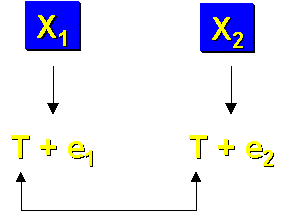If the measure X is reliable, it should be about the same when measured twice. This is because the only thing that the two observations have in common is their true scores, T. Error scores (e1 and e2) have different subscripts indicating that they are different values. This means that the two observed scores, X1 and X2 are related only to the degree that the observations share true score (assuming error score is random). Errors may lead to better or worse performance, but the true score would be the same on both observations (assuming that the T didn't change between the measurement occasions).
Statistically, reliability is a ratio or fraction, defined as:

(1)
\begin{align} \frac{true level on the measure}{the entire measure} \end{align}

Reliability isn’t a measure for an individual. It is a characteristic of a measure that's taken across individuals. The easiest way to restate the definition above in terms of a set of observations is to speak of the variance of the scores.

(2)
\begin{align} \frac{var(T)}{var(X)} \end{align}

The denominator of the reliability ratio can be easily calculated as the variance of the set of scores we observed. However, true scores cannot be seen in actuality and hence, reliability cannot be computed because the variance of the true scores cannot be calculated.
The best way is to estimate it. Recalling the two observations, X1 and X2, it is assumed (using true score theory) that these two observations would be related to each other to the degree that they share true scores. This estimation can be done by calculating the correlation between X1 and X2.

(3)
\begin{align} \frac{covariance(X1,X2)}{sd(X1).sd(X2)} \end{align}

We can see that the covariance is an indicator of the variability of the true scores because the true scores in X1 and X2 are the only thing the two observations share. So, the numerator is essentially an estimate of var(T) in this context. As for the denominator, it is expected that these two sd values would be the same (it is the same measure being taken) and that this is essentially the same thing as squaring the standard deviation for either observation. So, the denominator of the equation becomes the variance of the measure (or var(X)). Hence, the correlation between two observations of the same measure is an estimate of reliability.
However, recalling that because X = T + e, the denominator can be substituted as:

(4)
\begin{align} \frac{var(T)}{var(T) + var(e)} \end{align}

A perfectly reliable measure, the equation would reduce to:
have had factors affecting his ability to perform, contributing to errors in measurement that make the student's observed ability appear lower than their true or actual ability.If the measure X is reliable, it should be about the same when measured twice. This is because the only thing that the two observations have in common is their true scores, T. Error scores (e1 and e2) have different subscripts indicating that they are different values. This means that the two observed scores, X1 and X2 are related only to the degree that the observations share true score (assuming error score is random). Errors may lead to better or worse performance, but the true score would be the same on both observations (assuming that the T didn't change between the measurement occasions).
Statistically, reliability is a ratio or fraction, defined as:

(5)
\begin{align} \frac{true level on the measure}{the entire measure} \end{align}

Reliability isn’t a measure for an individual. It is a characteristic of a measure that's taken across individuals. The easiest way to restate the definition above in terms of a set of observations is to speak of the variance of the scores.

(6)
\begin{align} \frac{var(T)}{var(X)} \end{align}

The denominator of the reliability ratio can be easily calculated as the variance of the set of scores we observed. However, true scores cannot be seen in actuality and hence, reliability cannot be computed because the variance of the true scores cannot be calculated.
The best way is to estimate it. Recalling the two observations, X1 and X2, it is assumed (using true score theory) that these two observations would be related to each other to the degree that they share true scores. This estimation can be done by calculating the correlation between X1 and X2.

(7)
\begin{align} \frac{covariance(X1,X2)}{sd(X1).sd(X2)} \end{align}

We can see that the covariance is an indicator of the variability of the true scores because the true scores in X1 and X2 are the only thing the two observations share. So, the numerator is essentially an estimate of var(T) in this context. As for the denominator, it is expected that these two sd values would be the same (it is the same measure being taken) and that this is essentially the same thing as squaring the standard deviation for either observation. So, the denominator of the equation becomes the variance of the measure (or var(X)). Hence, the correlation between two observations of the same measure is an estimate of reliability.
However, recalling that because X = T + e, the denominator can be substituted as:

(8)
\begin{align} \frac{var(T)}{var(T)} \end{align}

and reliability = 1. For a perfectly unreliable measure, there is no true and the equation would reduce to:

(9)
\begin{align} \frac{0}{var(e)} \end{align}

and the reliability = 0. Thus reliability will always range between 0 and 1. The value of a reliability estimate is the proportion of variability in the measure attributable to the true score. A reliability of 0.8 means the variability is about 80% true ability and 20% error.

## Types of Reliability

It's not possible to calculate reliability exactly. Instead, it has to be estimated, and this is always an imperfect endeavor. There are four general classes of reliability estimates, each of which estimates reliability in a different way. They are:

1. Inter-Rater or Inter-Observer Reliability. Used to assess the degree to which different raters/observers give consistent estimates of the same phenomenon.
2. Test-Retest Reliability. Used to assess the consistency of a measure from one time to another.
3. Parallel-Forms Reliability. Used to assess the consistency of the results of two tests constructed in the same way from the same content domain.
4. Internal Consistency Reliability. Used to assess the consistency of results across items within a test.

### Inter-Rater or Inter-Observer Reliability

Whenever humans are used as a part of a measurement procedure, reliability or consistencies of results are questionable. People tend to be inconsistent – are easily distracted, get tired of doing repetitive tasks, daydream, misinterpret.
To determine whether two observers are consistent in their observations, inter-rater reliability outside of the context of the measurement in the study should be established. It's best to do this as a side study or pilot study. If the study goes on for a long time, inter-rater reliability should be re-established from time to time to assure that the raters aren't changing.
There are two major ways to estimate inter-rater reliability. For categorical data, the percent of agreement between the raters could be calculated. Though crude, it does give an idea of how much agreement exists, and it works no matter how many categories are used for each observation.
The other major way to estimate inter-rater reliability is appropriate when the data is continuous, where the correlation between the ratings of the two observers is calculated. This would give an estimate of the reliability or consistency between the raters.
This type of reliability could be considered as "calibrating" the observers. There are other things that could be done to encourage reliability between observers. For instance weekly "calibration" meetings could be held, where observers would discuss why they chose specific values they did. If there were disagreements, the observers would discuss them and attempt to come up with rules for deciding when they would give a rating on a specific item. Although this will not be an estimate of reliability, it will go a long way towards improving the reliability between raters.

### Test-Retest Reliability

Test-retest reliability is used when the same test is administered to the same sample on two different occasions. This approach assumes that there is no substantial change in the construct being measured between the two occasions. The amount of time allowed between measures is critical, as the correlation between the two observations will depend in part by how much time elapses between measurements. The shorter the time gap, the higher the correlation; the longer the time gap, the lower the correlation. Since this correlation is the test-retest estimate of reliability, considerably different estimates could be obtained depending on the interval.### Parallel-Forms Reliability

In parallel forms reliability, two parallel forms have to be created. One way to accomplish this is to create a large set of questions that address the same construct and then randomly divide the questions into two sets. Both instruments are administered to the same sample of people. The correlation between the two parallel forms is the estimate of reliability. A major problem with this approach is that many items that reflect the same construct have to be generated, which isn’t easy. Furthermore, this approach makes the assumption that the randomly divided halves are parallel or equivalent. This is sometimes not the case.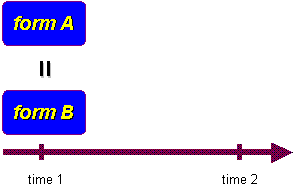The parallel forms approach is similar to the split-half reliability described below. The major difference is that parallel forms are constructed so that the two forms can be used independent of each other and considered equivalent measures. For instance, we might be concerned about a testing threat to internal validity. If Form A was used for the pretest and Form B for the posttest, that problem is minimized. With split-half reliability, there is only a single measurement instrument and randomly split halves are only developed for purposes of estimating reliability.

### Internal Consistency Reliability

In internal consistency reliability estimation, a single measurement instrument is administered to a group of people on one occasion to estimate reliability. In effect, reliability of the instrument is judged by estimating how well the items that reflect the same construct yield similar results, and how consistent the results are for different items for the same construct within the measure. There are a wide variety of internal consistency measures that can be used.

#### * Average Inter-item Correlation

The average inter-item correlation uses all of the items on the instrument that are designed to measure the same construct. First, the correlation between each pair of items are computed, as illustrated in the figure. The average inter-item correlation is simply the average or mean of all these correlations.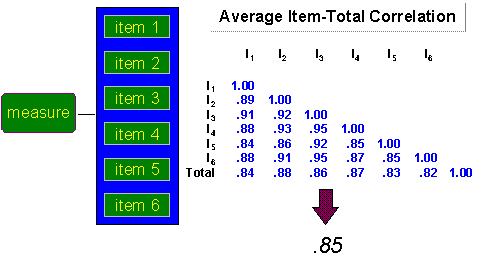#### * Average Item-total Correlation

This approach also uses the inter-item correlations. In addition, total score for the six items are computed and used as a seventh variable in the analysis. The figure shows the six item-to-total correlations at the bottom of the correlation matrix.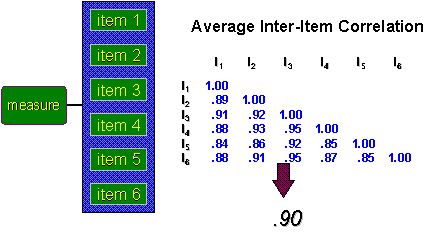#### * Split-Half Reliability

In split-half reliability, all items that purport to measure the same construct are randomly divided into two sets. The entire instrument is administered to a sample of people and the total score for each randomly divided half is calculated. The split-half reliability estimate, as shown in the figure, is simply the correlation between these two total scores.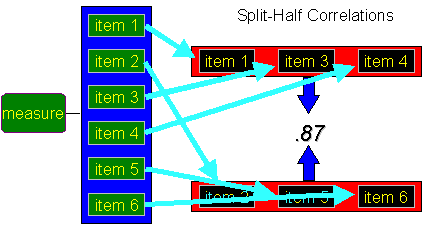#### * Cronbach's Alpha (α)

Cronbach's Alpha is the mathematical equivalent to the average of all possible split-half estimates, although that's not how it is computed. It doesn't mean that each time a new sample is measured. Instead, we all split-half estimates are calculated from the same sample. As all the samples on each of the six items are measured, all that is to be done is have the computer analysis do the random subsets of items and compute the resulting correlations. The figure shows several of the split-half estimates for the six item example and lists them as SH with a subscript. Note that although Cronbach's Alpha is equivalent to the average of all possible split half correlations, it would never actually be calculate it that way.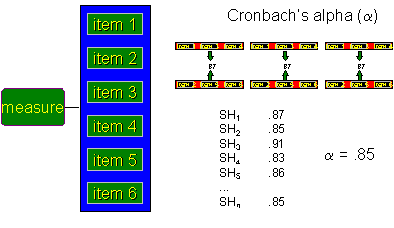## Comparison of Reliability Estimators

Each of the reliability estimators has certain advantages and disadvantages. Inter-rater reliability is one of the best ways to estimate reliability when a measure is an observation. However, it requires multiple raters or observers. As an alternative, the correlation of ratings of the same single observer repeated on two different occasions could be used. For example, videotapes of child-mother interactions are collected and had a rater code the videos for how often the mother smiled at the child. To establish inter-rater reliability a sample of videos could be taken and have two raters code them independently. To estimate test-retest reliability a single rater code could be carried out on the same videos on two different occasions. Inter-rater approach could be used especially if there is a team of raters and they need to yield consistent results. If a suitably high inter-rater reliability was obtained, they could be justified to work independently on coding different videos. Test-retest approach could be used when a single rater is used. On the other hand, in some studies it is reasonable to do both to help establish the reliability of the raters or observers.

The parallel forms estimator is typically only used in situations where two forms are used as alternate measures of the same thing. Both the parallel forms and all of the internal consistency estimators have one major constraint – multiple items have to be designed to measure the same construct. This is relatively easy to achieve in certain contexts like achievement testing, but for more complex or subjective constructs this can be a real challenge. If there are a lot of items, Cronbach's Alpha tends to be the most frequently used estimate of internal consistency.
The test-retest estimator is especially feasible in most experimental and quasi-experimental designs that use a no-treatment control group. In these designs there is always a control group that is measured on two occasions (pretest and posttest). The main problem with this approach is that there isn’t any information about reliability until the posttest is collected. If the reliability estimate is low, the test is sunk.

Each of the reliability estimators will give a different value for reliability. In general, the test-retest and inter-rater reliability estimates will be lower than the parallel forms and internal consistency ones because they involve measuring at different times or with different raters. Since reliability estimates are often used in statistical analyses of quasi-experimental designs (e.g., the analysis of the nonequivalent group design), the fact that different estimates can differ considerably makes the analysis even more complex.

## Reliability & Validity

Reliability and validity are, in fact, related to each other. Imagine the center of the target as the concept that is being measured. For each person you measured, a shot is taken at the target. Perfect measurement means hitting the centre of the target. Otherwise it’s a miss.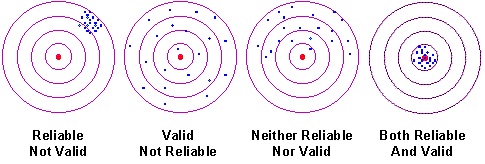The figure above shows four possible situations. In the first one, the target is hit consistently, but misses the center. That is, the wrong values for all respondents are consistently and systematically measured. This measure is reliable, but not valid (that is, it's consistent but wrong). The second shows hits that are randomly spread across the target. The target centre is seldom hit but, on average, the right answer for the group is obtained. In this case, there is a valid but inconsistent group estimate. Here, is it clear that reliability is directly related to the variability of measure. The third scenario shows a case where the hits are spread across the target but are consistently missing the center, a measure that is neither reliable nor valid. Finally, in the last scenario, the target center is consistently hit. The measure is both reliable and valid.

Reference:
Research Methods Knowledge Base, www.socialresearchmethods.net/kb/reltypes.php

page revision: 5, last edited: 08 Jun 2007 09:55
Unless otherwise stated, the content of this page is licensed under Creative Commons Attribution-Share Alike 2.5 License.